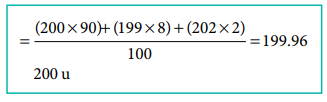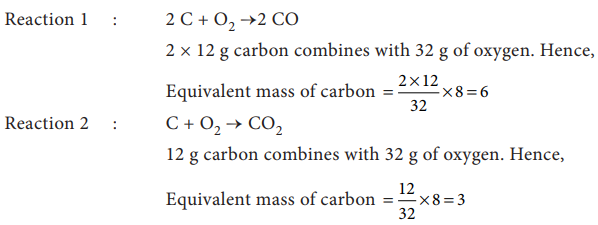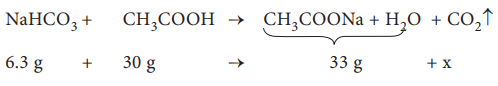# UNIT 1: Basic Concepts of Chemistry and Chemical Calculations - Online Test

Q1. 40 ml of methane is completely burnt using 80 ml of oxygen at room temperature The volume of gas left after cooling to room temperature is
Explaination / Solution:

CH4(g) + 2O2(g) → CO2 (g)+ 2 H2O (l)Since the product was cooled to room temperature, water exists mostly as liquid. Hence, option (a) is correct

Q2. An element X has the following isotopic composition 200X = 90 %, 199X = 8 % and 202X = 2 %. The weighted average atomic mass of the element X is closest to
Explaination / Solution:Q3.

Assertion Two mole of glucose contains 12.044 × 1023 molecules of glucose

Reason : Total number of entities present in one mole of any substance is equal to 6.02 × 1022

Explaination / Solution:

Correct reason: Total number of entities present in one mole of any substance is equal to 6.022 ×1023.

Q4. Carbon forms two oxides, namely carbon monoxide and carbon dioxide. The equivalent mass of which element remains constant?
Explaination / Solution:Q5. The equivalent mass of a trivalent metal element is 9 g eq-1 the molar mass of its anhydrous oxide is
Explaination / Solution:

Let the trivalent metal be M3+

Equivalent mass = mass of the metal / valance factor

9 g eq-1 = mass of the metal / 3 eq

Mass of the metal = 27 g

Oxide formed M2O3 ;

Mass of the oxide = (2 x 27) + (3 x 16)

= 102 g

Q6. The number of water molecules in a drop of water weighing 0.018 g is
Explaination / Solution:

Weight of the water drop  =0.018 g

No. of moles of water in the drop=Mass of water / molar mass

=  0.018 / 18 = 10-3 mole

No of water molecules present in 1 mole of water= 6.022 x 1023

No. water molecules in one drop of water (10-3 mole)= 6.022 x 1023x 10-3

=6.022 x 1020

Q7. 1 g of an impure sample of magnesium carbonate (containing no thermally decomposable impurities) on complete thermal decomposition gave 0.44 g of carbon dioxide gas. The percentage of impurity in the sample is
Explaination / Solution:
No Explaination.

Q8. When 6.3 g of sodium bicarbonate is added to 30 g of acetic acid solution, the residual solution is found to weigh 33 g. The number of moles of carbon dioxide released in the reaction is
Explaination / Solution:The amount of CO2 released, x = 3.3 g

No. of moles of CO2 released = 3.3 / 44 = 0.075 mol

Q9. When 22.4 litres of H2 (g) is mixed with 11.2 litres of Cl2 (g), each at 273 K at 1 atm the moles of HCl (g), formed is equal to
Explaination / Solution:

H2(g) + Cl2(g) → 2 HCl (g)Q10. Hot concentrated sulphuric acid is a moderately strong oxidising agent. Which of the following reactions does not show oxidising behaviour?
Explaination / Solution: